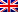# Help with the decision

Contenus

## Help with the decision

Any industrial problem consists, under certain conditions, of maximizing profit or minimizing expenditure. In this context, profit and expenditure do not always refer to a monetary variable, it can also be expressed by time, distance or others.

Very often, the problem is stated in a crude manner, that is to say by a text or a specification. The manufacturer was not an expert in the field of writing a mathematical problem, the specifications include all kinds of data, useful or not for its modeling.

Even in the context where you yourself are the sponsor, you may not know the extent of your problem, and you discover over the course of the water the various constraints and variables to be faced.

Another problem arises once the mathematical modeling carried out: what computer tool was used to solve this problem? what algorithm Choose ? his simulation? her complexity ? its optimality?

Building and solving an industrial problem therefore requires rigor, flexibility of mind and following a precise modeling process.

## Optimization and decision support

A problem D is deciding whether the answer is binary: Yes or No. We denote Yes (D) all the instances to which we answer Yes.

Consider the following decision problem: let a graph G weighted, is there a tree covering? Yes(D) = {non-cyclic connected subgraph of G}. The problem: existence of a tree covering of weight ≤ k is also a decision problem. The optimization problem is to find the value k such that it is minimal.

In order to better understand the two concepts, we will take an example: You wish to take a tour of Europe, by visiting a certain number of cities within a period of 6 months. In addition, you want to stay a certain period of time in each place in order to be able to visit the tourist areas and admire the landscape.

This kind of problem has different ways of being modeled depending on what you want to do: be the fastest, favor densely touristy areas, etc. A decision must be selected from among a set of possible decisions so as to optimize the chosen criterion.

Modeling includes a search for minimum or maximum, so it is a optimization. Decision support problems all contain the following three points:

• The type of decision: what we want to do (here we are looking for an optimization)
• Possible decisions: what can be done (the definition field)
• The selection criterion: how one chooses (the modeling of the problem).

The studied problem is placed in a certain context which will be translated into parameters. All the relationships between these are shown in the model. The latter can either take the form of a mathematical model or a graph.

Modeling is only a schematic representation of the problem, only the elements considered relevant are retained in the construction of the decision. It proceeds by simplifications and omissions.

The environment of the model can also play a role. Whether deterministic or with uncertainty, it is present via laws of probability, stochastics, etc. within constraints.

The selection criterion can lead to different solutions depending on the parameter put forward. In some cases, the model presents only one selection criterion, this is called operational research.

All models consist of three basic components:

• Result variables are outputs. The reflect the level of efficiency of the system. They are dependent variables.
• Decision variables describe alternative actions.
• Uncontrollable variables are factors that affect the outcome but are not under the control of the decision maker. Either these factors are fixed or they may vary.

Components are linked together by expressions math in a framework of quantitative models. A principle of choice is a criterion that describes the acceptability of an approach solution.

A model can be a normative model or a descriptive model. In the first, the solution chosen is clearly the best of all possible alternatives. To find it, we must examine all the alternatives and prove that the one that is selected is indeed the best (we speak of optimization). Descriptive models study alternative actions under different input and process configurations. All the alternatives are not verified, only a given set is.

## Solution and decision support

Once the model has been created and a solution has been found, it is important to analyze it in order to validate the model. The latter was only a schematic representation of the problem, it may not be suitable for the intended purpose. A solution highlights the validity of the decision choices and the choices of the model. Only the decision-maker / sponsor can validate the approach taken.

The diagram of the decision support process is as follows:FRFRENES